# Triangle

Determine if it is possible to construct a triangle with sides 28 31 34 by calculation.

Result

#### Solution:

$a+b>c \land b+c>a \land a+c > b \ \\ 28 + 31 > 34 \ \\ 31 + 34 > 28 \ \\ 28 + 34 > 31$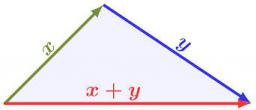Our examples were largely sent or created by pupils and students themselves. Therefore, we would be pleased if you could send us any errors you found, spelling mistakes, or rephasing the example. Thank you!

Leave us a comment of this math problem and its solution (i.e. if it is still somewhat unclear...):Be the first to comment!## Next similar math problems:

1. TriangleProve whether you can construct a triangle ABC, if a=9 cm, b=6 cm, c=10 cm.
2. Triangle P2Can triangle have two right angles?
3. 3-bracket 2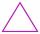May be the smallest angle in the triangle greater than 40°?
4. Circles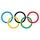For the circle c1(S1; r1=146 cm) and c2(S2; r2 = 144 cm) is distance of centers |S1S2| = 295 cm. Determine the distance between the circles.
5. CagesHonza had three cages (black, silver, gold) and three animals (guinea pig, rat and puppy). There was one animal in each cage. The golden cage stood to the left of the black cage. The silver cage stood on the right of the guinea pig cage. The rat was in the
6. Compare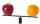Compare with characters >, <, =: 85.57 ? 80.83
7. Flood waterFlood waters in some US village meant that the homes had to evacuate 364 people. 50 of them stayed at elementary schools, 59 them slept with their friends and others went to relatives. How many people have gone to relatives?Added together and write as decimal number: LXVII + MLXIV
9. Angles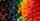Which of those angles are obtuse?
10. Plane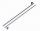On how many parts divide plane 6 parallels?
11. Six te 2If 3t-7=5t, then 6t=
12. CakesOn the bowl were a few cakes. Jane ate one-third of them, Dana ate a quarter of those cakes that remained. a) What part (of the original number of cakes) Dana ate? b) At least how many cakes could be (initially) on thebowl?
13. One frame5 picture frames cost € 12 more than three frames. How much cost one frame?
14. Valid numberRound the 453874528 on 2 significant numbers.
15. Eq1Solve equation: 4(a-3)=3(2a-5)
16. Math classificationIn 3A class are 27 students. One-third got a B in math and the rest got A. How many students received a B in math?
17. The resultHow many times I decrease the number 1632 to get the result 24?